## Friday, May 4, 2018

### Curving pitches, kicks, and shots…

So… In baseball and softball, many batters batters hate the curveball… Because it curves…
NOTE: The physics of this is true for ALL sports where a ball moves through the air… tennis, golf, ping pong, volleyball, soccer (bend it like Becker)…
Now, the curve in question is in addition to the fact that it is moving from the picture to the batter as a projectile which automatically means that it's up and down is changing at the same time it moves closer and closer to the batter.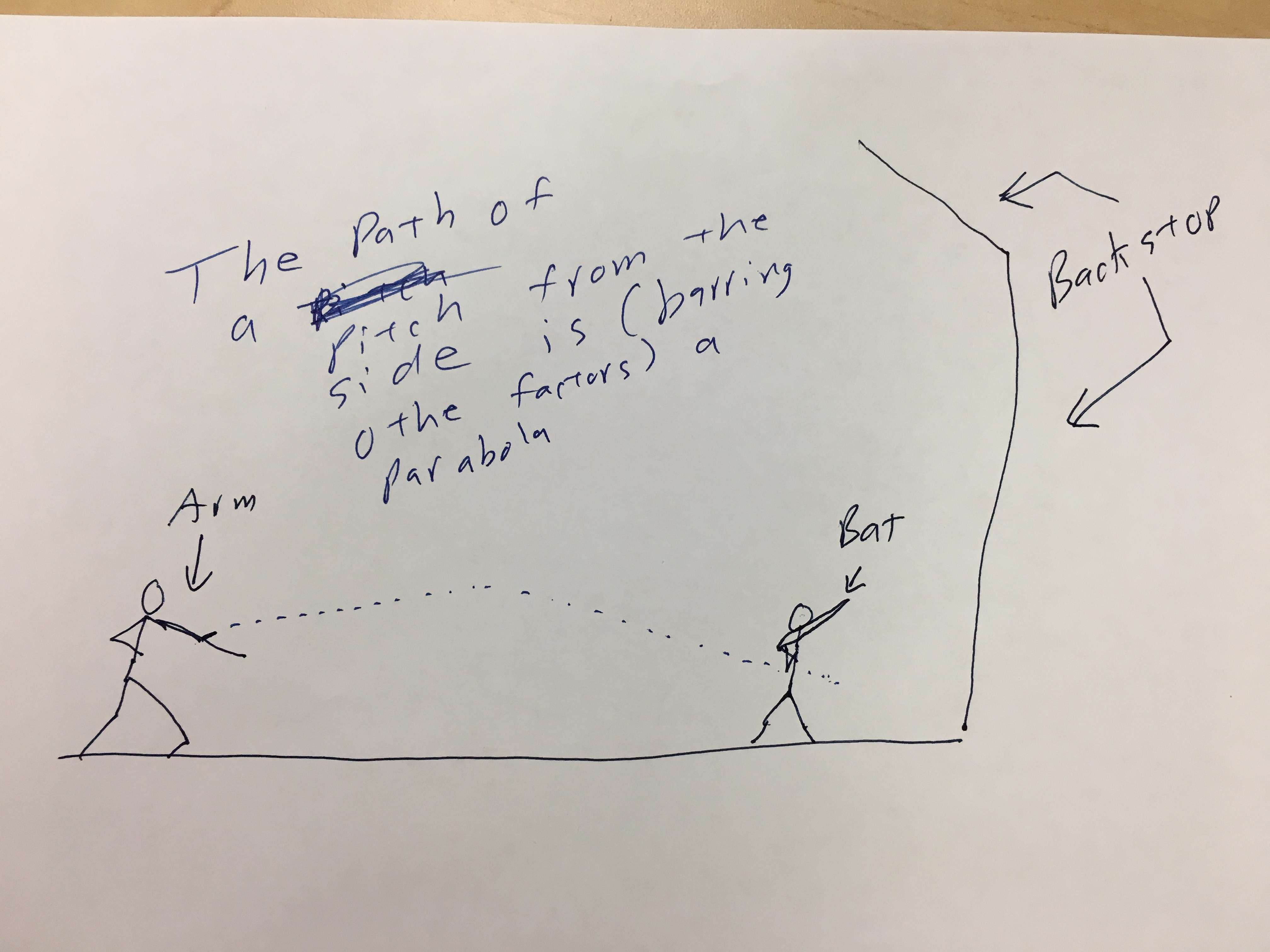Imagine slow-pitch softball or a lobbed pitch… In the extreme, think about soft toss… The ball moves up and down as it approaches the strike zone.
So, every pitch regardless of type is, from the moment it is released affected by these three forces:
1. Gravity pulls the ball down toward the center of the earth.
2. There is a tiny, tiny buoyant force that is far far less than the force of gravity.
3. There is air resistance (friction) that opposes the motion of they ball and which is always directly directly opposite the direction of the instantaneous velocity.
Now, let's talk about rotation! Because, except for a knuckle ball, pitches rotate…
I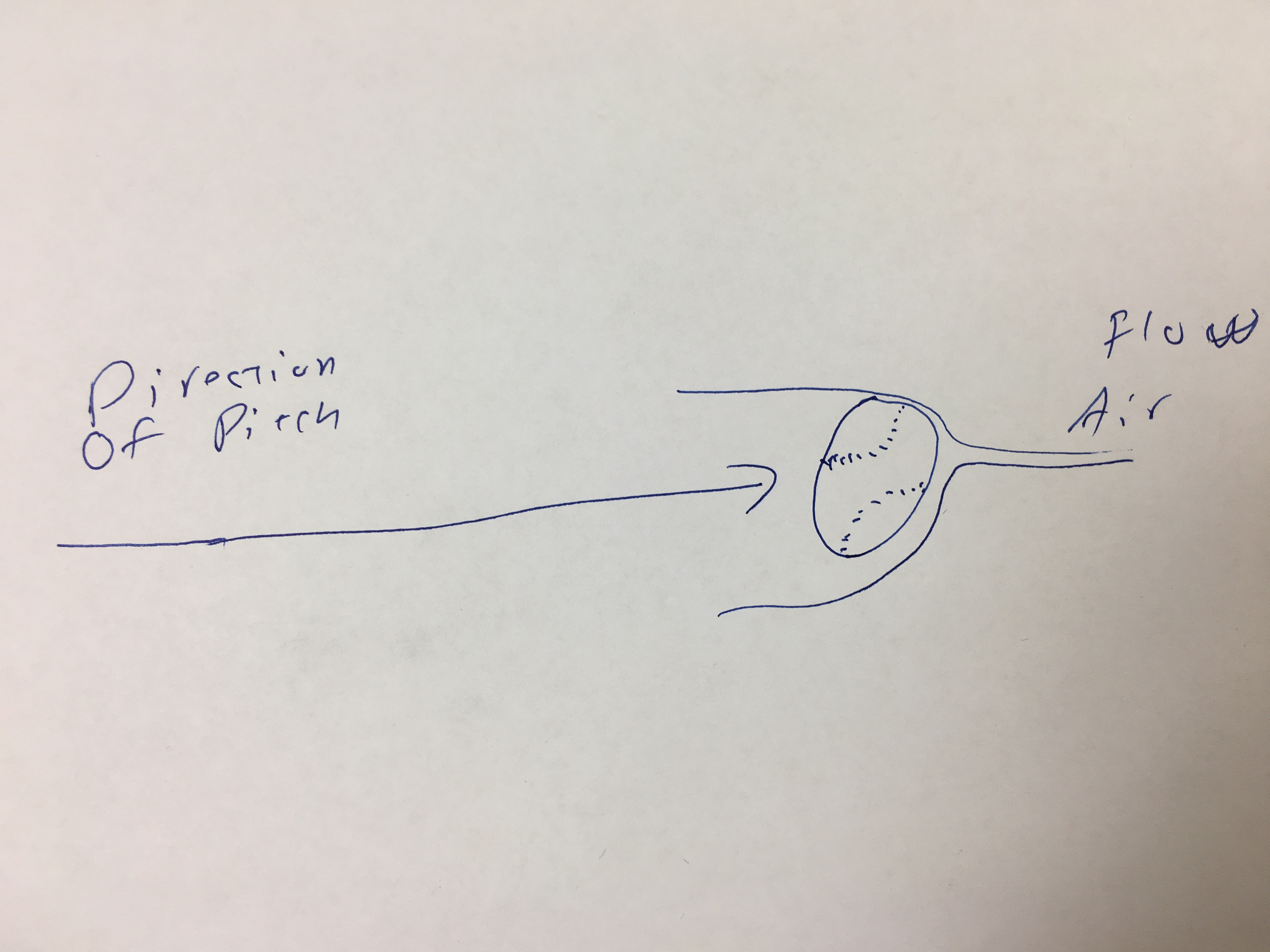magine a ball spinning on an axil, not moving. When the ball spins, the air around it is "drug" in the direction of the spin. Now, if you add in the movement of the ball, something happens!
First if the ball is thrown and spin is ignored, you get an object moving through the air. The air flows around the ball equally. Nothing interesting there…
However, if you account for the spinning of the ball… and the air that is being drug around it by the spin, you start to see something.

When air moves faster, is gets stretched out. There are fewer molecules of air in the same space. From the gas laws, it is known that if you have fewer molecules in the same space, the pressure is lower (given constant temperature, which can be assumed over the diameter of a baseball or softball).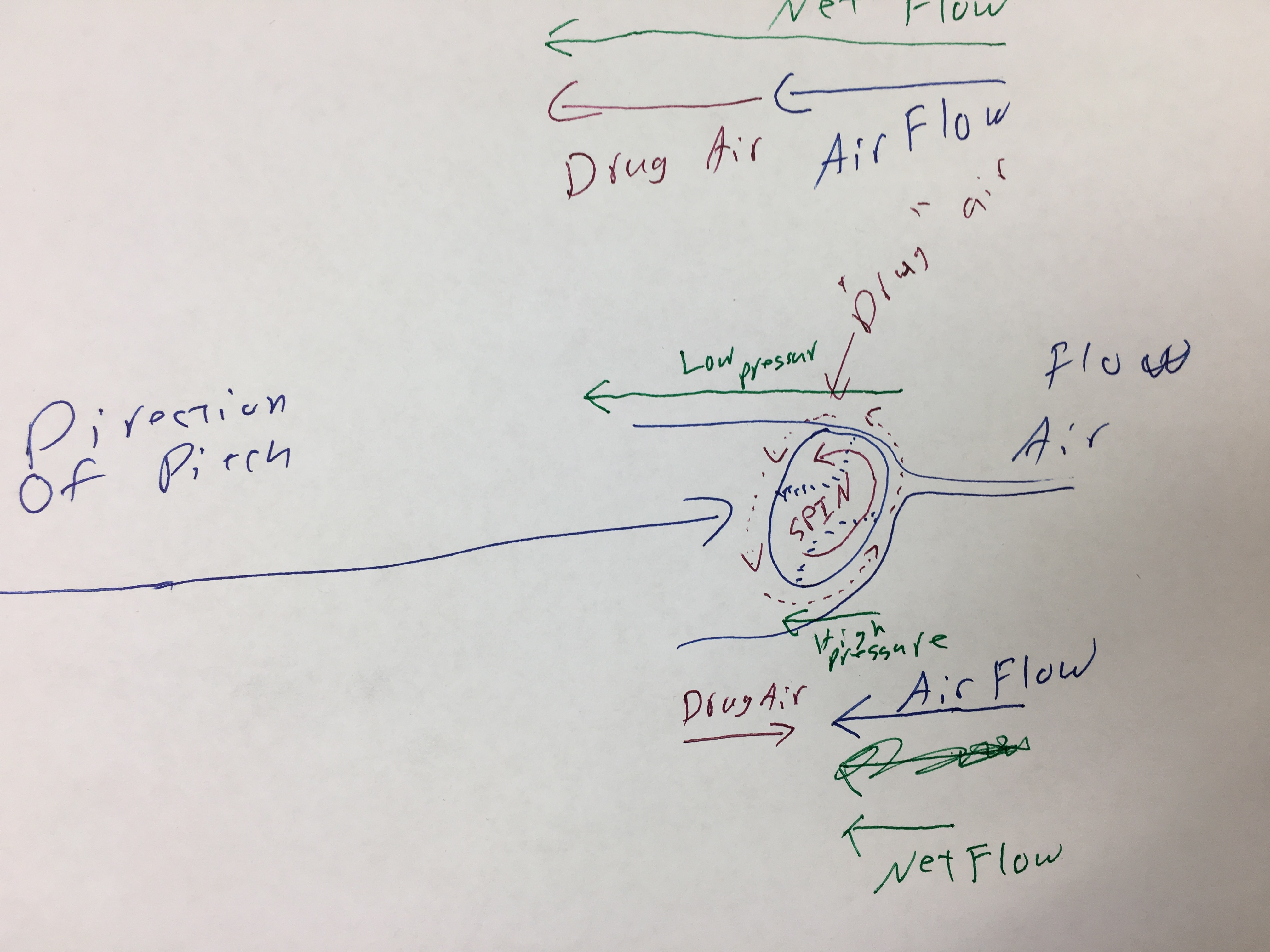So, the spinning ball drags the air around it, AND those moving molecules of air interact with the moving air as the pitch moves through the air.  Where the drug air is going the same way as the air flow, the molecules move faster, but where the drug air is going in the opposite direction, the air flow slows down.
The result is that one side of the ball has high pressure and the other side has low pressure. The difference in pressure creates a force. The air pressure difference pushes the ball form the high pressure region to the low pressure region.
That is to say there is a force acting in the direction of the low pressure. Given Newton's Second Law, it is known that F=ma, so where there is a net force, the ball must accelerate (change velocity) in the direction of the force.
Thus, the rate of acceleration can be found based on some function of the difference in pressure (F) divided by the mass of the ball:
a = ƒ(p1 and p2)/m
So, what can a pitcher do to make the ball curve? Well… you can't control the mass of the ball. You can control, however the spin.
Since the air moving around the ball due to it moving is the same on all sides, the spin accounts for the difference in pressure. Both p1 and p2 are affected by the spin
T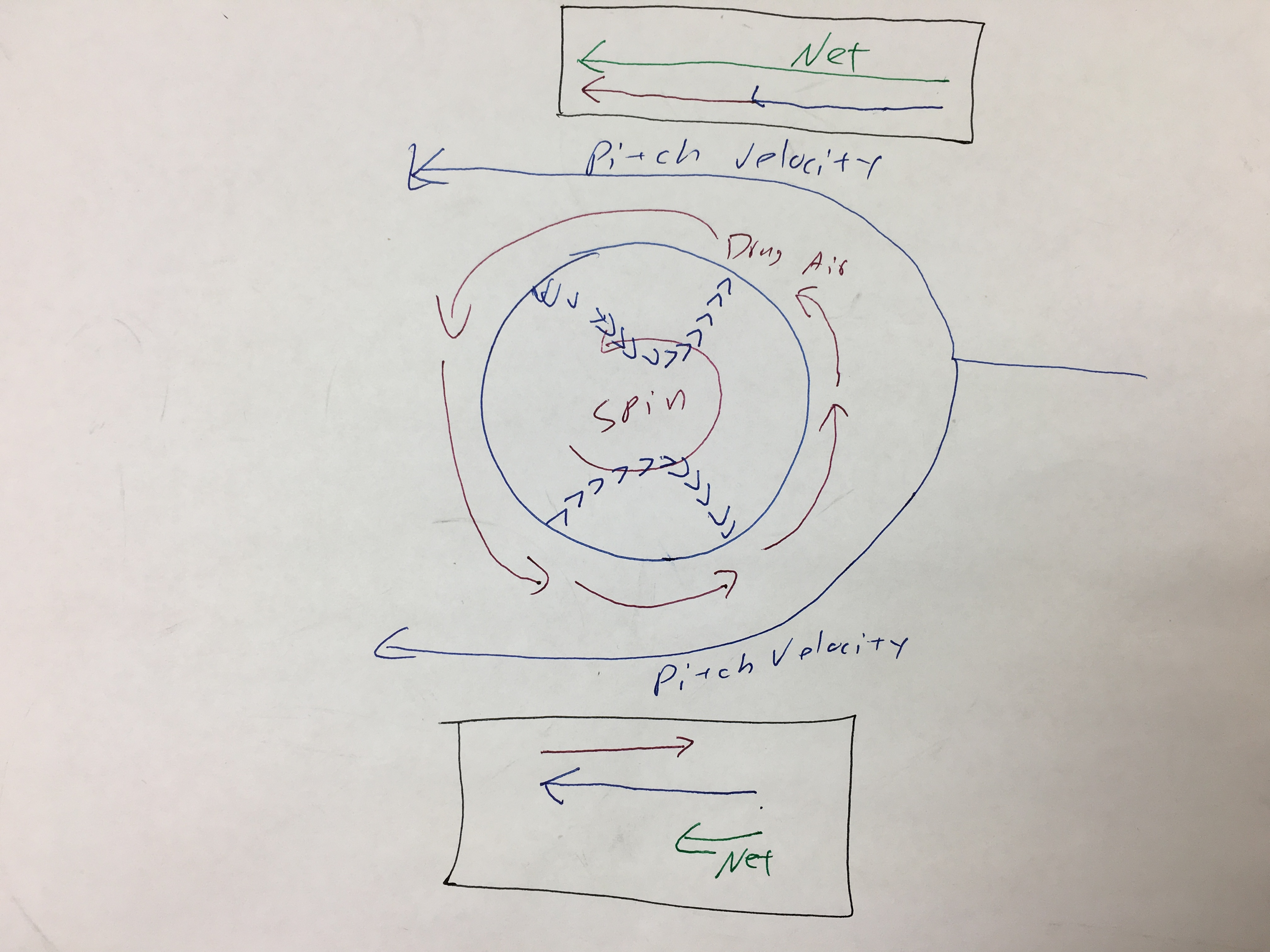he net air flow is on one side pitch velocity + drug air.
The net air flow on the other side is pitch velocity - drug air.
Thus, since the difference in pressure is a result of the DIFFERENCE in the net velocities of the air on opposite sides of the pitch path, the more spin, the bigger the difference in pressure.
AND, then, the bigger the difference in pressure (back to the math)…
a = ƒ(p1 and p2)/m
…the greater the rate of acceleration.
By controlling the spin allows a pitcher to control where the high and low pressure zones are. The ball will break into the low pressure zone.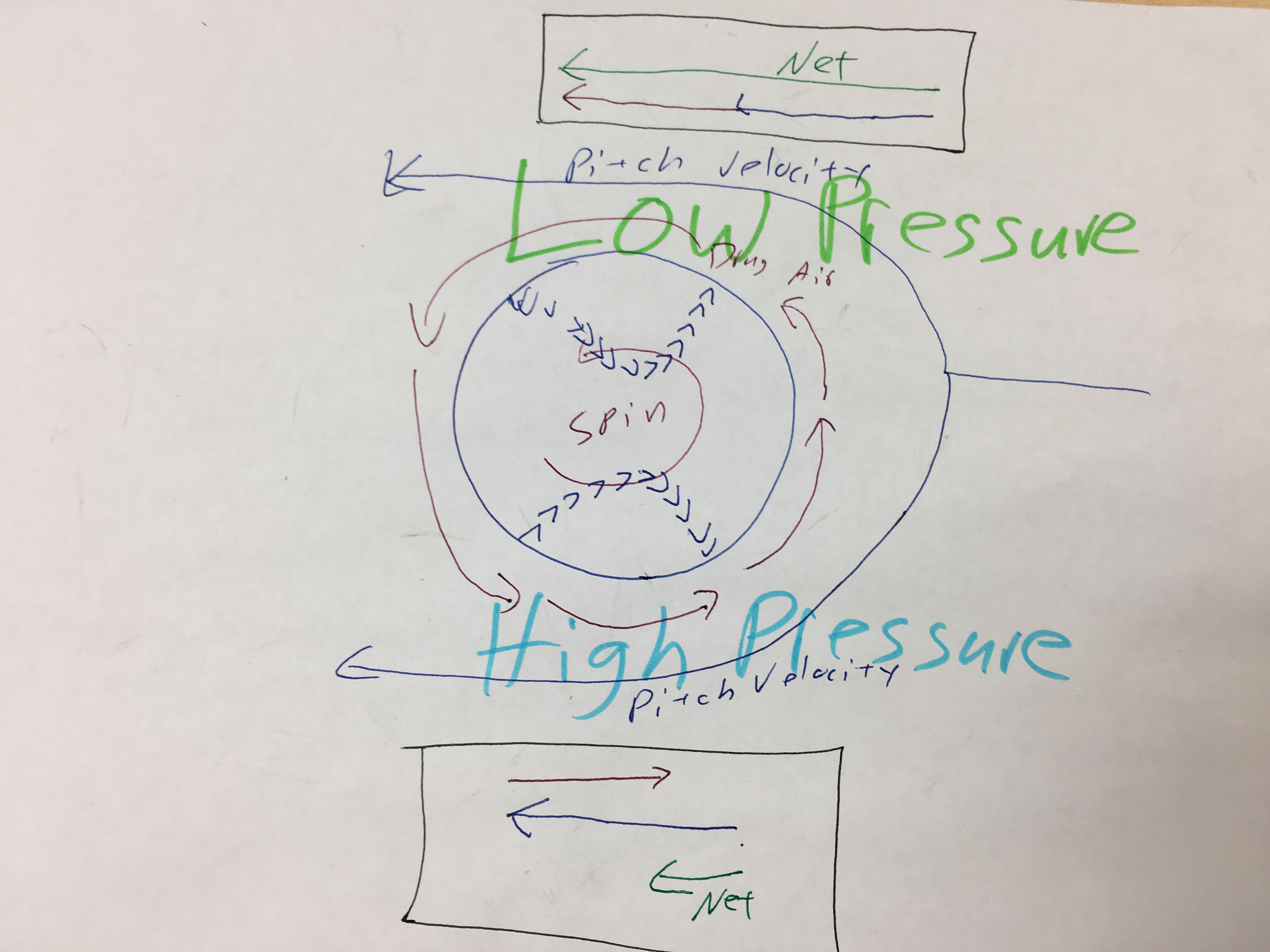A pitch, then, with backspin, will dive. If the rotation is the other way (e.g. a good overhand throw), the ball will actually float some and the effect of gravity will be lessoned.
The LESS the axis of rotation aligns with the path of the ball, the GREATER the affect of the spin on the ball's path.
Conversely, if the axis of rotation aligns with the path of the ball's movement, then the affect of the spin is zero. Think of a spiral football pass. IF the pass/punt spirals properly, the affect of the spin is zero.
An overhand fastball rotates back toward the pitcher. Thus, there is low pressure on top of the ball.
An underhand fastball (or a topspin tennis shot) rotates forward toward the batter. Thus, there is low pressure on the bottom of the ball.
Cutters, sliders, etc have various spins that move the low pressure zone to different places on the ball.
CONCLUSION:
1.) Since the rate at which a ball's velocity changes can be found by
a = ƒ(p1 and p2)/m
and since the difference in pressures is caused by the spin, then changing the direction and rate of the spin will change the direction and rate of the acceleration (of the break).
2.) Increasing the rate of spin increases the difference in pressures. The greater the difference in pressure, the more the ball with break (accelerate).

1.) The diameter of the ball affects the velocity of the spinning surface. Thus, two balls of the same mass spinning at the same rate, but having different diameters will break differently. For example, a huge beachball with the same mass as a volleyball will curve more with the same spin.

## Tuesday, May 1, 2018

### Friction: A Force That Opposes Motion

Newton's Second Law leads to the formula that connects force, mass, and acceleration:

F = ma

Earlier, it was explained that there are only four fundamental forces, but that every other force that is easily observed is derived from them. Thus, friction can be understood as a force which arises due to the interaction of the atoms between two objects when they are in contact. The strong, weak, and electromagnetic forces work such that there is a resistive force when one object slides across another.

A resistive force (also know as oppositional force) works in the opposite direction of the active forces, such as pushing or pulling something.

Before working with friction, there are two more concepts that need to be understood:

### Weight

Weight is the force created by gravity and its magnitude can be found by multiplying the mass of an object by the rate at which gravity accelerates things. On earth, for introductory physics students,. the value for acceleration due to gravity of 9.8 (m/s)/s or 9.81 (m/s)/s is commonly used.

In the metric system, the Newton is the unit for weight. The pound is also a unit of weight*.

The direction for weight is always "down." That means that (when on earth) weight always acts in direction that is perpendicular to the horizon and toward the center of the earth.

### Normal ForceThe concept of Normal Force is easy to understand. Calculating the normal force (which is usually indicated as Fn) requires using trigonometry EXCEPT when the surface on which an object slides is level (has an angle to the horizon of zero).

The formal force (Fn) is defined as the component of the weight (mg) of an object that is perpendicular to a surface. When the surface is level ALL of the normal force is perpendicular to the surface. That makes things easy. Thus, the normal force IS THE WEIGHT, but this is ONLY true with the surface is level (has and angle to the horizon of zero).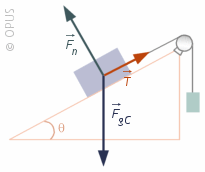When the surface is NOT level, then, math…

Fn is labeled, and it is clear that it pushing the box in a direction that is perpendicular to the surface of the inclined plane.

### Note that F3 is perpendicular to the horizon. That would mean that F3 is the weight or is found as m•g where m is the mass and g is acceleration due to gravity.Force of Friction

There are two types of friction. One is when an object is already sliding. One is when the object is at rest. They are cleverly named sliding friction and static friction.

Sliding friction is sometimes indicated as fk where the k stands for kinetic (which means moving) and static friction is indicated as fs.

NOTE: in this nomenclature, the fi is lowercase. the k and the s may or may not be subscripted, depending on the type-setting process.

Intuition will tell you that pushing something over ice is easier than pushing it over asphalt. There therefore must be some way to indicate the degree of slipperiness an object has (or stickiness).

There is. It is called the coefficient of friction. And there are two sets of coefficients: one for each type of friction. Sliding and static coefficients of friction are abbreviated as µk and µs respectively.

Here is a link that will allow you to look at coefficients of friction for various materials:

https://www.engineeringtoolbox.com/friction-coefficients-d_778.html

### Calculating the Force of Friction

So, using all those concepts, calculating the force of friction becomes pretty simple. Friction can be found by multiplying the normal force by the coefficient of friction:

fk = (Fn)(µk)

EXAMPLE

Clean dry steel sliding on steel has a coefficient of friction of μ = 0.78. If a block with a mass of 4 kg slides across, what is the force of friction.

First, calculate the normal force:

Fn = mg
Fn = (4)(9.8)
Fn = 39.2

Now, use Fn to find the force of friction.

fk = (Fn)(µk)
fk = 39.2 • 0.78

fk = 30.576 N

_________________
*The English system unit for mass is called the Slug and is approximated by taking the weight of something and dividing by 32. So a 110 pound person would have a mass of 3.4 slugs.